##### Quantum Physics For Dummies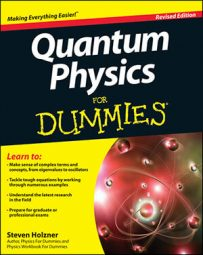What do Dirac notation and the Hermitian conjugate have in common? They help physicists to describe really, really big vectors. In most quantum physics problems, the vectors can be infinitely large — for example, a moving particle can be in an infinite number of states. Handling large arrays of states isn’t easy using vector notation, so instead of explicitly writing out the whole vector each time, quantum physics usually uses the notation developed by physicist Paul Dirac — the Dirac or bra-ket notation.

## Abbreviating state vectors as kets

Dirac notation abbreviates the state vector as a ket, like this: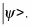For example, if you were trying to find the probabilities of what a pair of rolled dice was likely to show, you could write the state vector as a ket this way: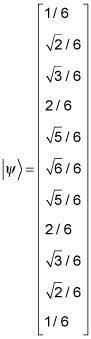Here, the components of the state vector are represented by numbers. More commonly, however, each component represents a function, something like this: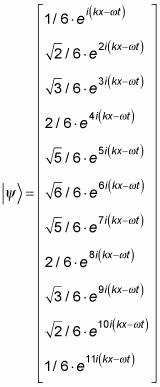You can use functions as components of a state vector as long as they’re linearly independent functions (and so can be treated as independent axes in Hilbert space). In general, a set of vectors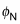in Hilbert space is linearly independent if the only solution to the following equation is that all the coefficients ai = 0: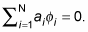That is, as long as you can’t write any one vector as a linear combination of the others, the vectors are linearly independent and so form a valid basis in Hilbert space.

## Writing the Hermitian conjugate as a bra

For every ket, there’s a corresponding bra. (The terms come from bra-ket, or bracket.) A bra is the Hermitian conjugate of the corresponding ket.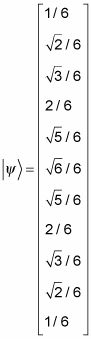The asterisk (*) symbol in the following equation means the complex conjugate. (A complex conjugate flips the sign connecting the real and imaginary parts of a complex number.) So the corresponding bra, which you write as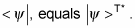The bra is this row vector: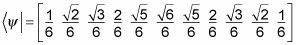Note that if any of the elements of the ket are complex numbers, you have to take their complex conjugate when creating the associated bra. For instance, if your complex number in the ket is a + bi, its complex conjugate in the bra is abi.

## Multiplying bras and kets

You can take the product of your ket and bra, denoted as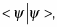like this: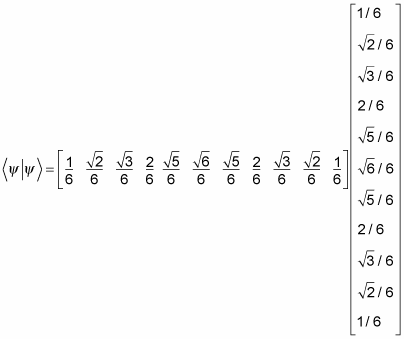This is just matrix multiplication, and the result is the same as taking the sum of the squares of the elements: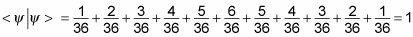And that’s the way it should be, because the total probability should add up to 1. Therefore, in general, the product of the bra and ket equals 1: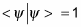If this relation holds, the ket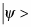is said to be normalized.